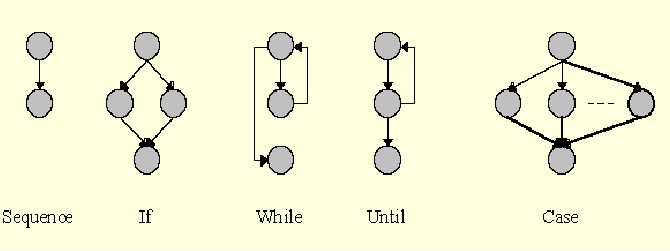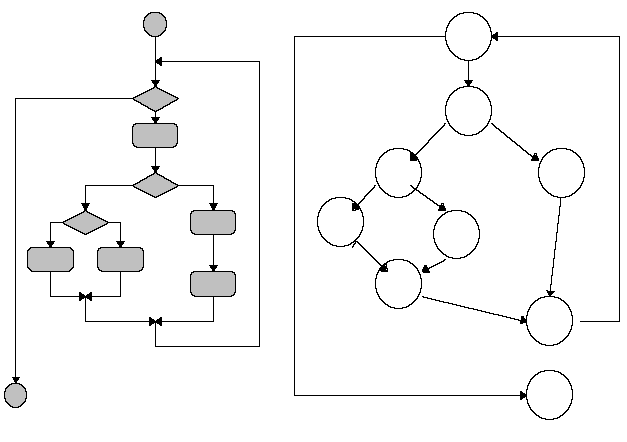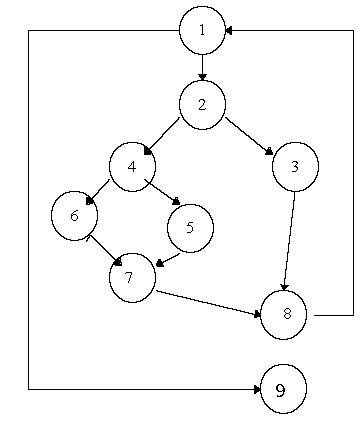## 1.3 Basis Path Testing

A testing mechanism proposed by McCabe.

Aim is to derive a logical complexity measure of a procedural design and use this as a guide for defining a basic set of execution paths.

Test cases which exercise basic set will execute every statement at least once.

### 1.3.1 Flow Graph Notation

Notation for representing control flowOn a flow graph:

• Arrows called edges represent flow of control
• Circles called nodes represent one or more actions.
• Areas bounded by edges and nodes called regions.
• A predicate node is a node containing a condition

Any procedural design can be translated into a flow graph.

Note that compound boolean expressions at tests generate at least two predicate node and additional arcs.

Example:### 1.3.2 Cyclomatic Complexity

The cyclomatic complexity gives a quantitative measure of the logical complexity.

This value gives the number of independent paths in the basis set, and an upper bound for the number of tests to ensure that each statement and both sides of every condition is executed at least once.

An independent path is any path through a program that introduces at least one new set of processing statements (i.e., a new node) or a new condition (i.e., a new edge)

 ``` 1: WHILE NOT EOF LOOP 2: Read Record; 2: IF field1 equals 0 THEN 3: Add field1 to Total 3: Increment Counter 4: ELSE 4: IF field2 equals 0 THEN 5: Print Total, Counter 5: Reset Counter 6: ELSE 6: Subtract field2 from Total 7: END IF 8: END IF 8: Print "End Record" 9: END LOOP 9: Print Counter ```Example has:

• Independent Paths:
1. 1, 9
2. 1, 2, 3, 8, 1, 9
3. 1, 2, 4, 5, 7, 8, 1, 9
4. 1, 2, 4, 6, 7, 8, 1, 9
• Cyclomatic Complexity of 4; computed using any of these 3 formulas:
1. #Edges - #Nodes + #terminal vertices (usually 2)
2. #Predicate Nodes + 1
3. Number of regions of flow graph.

Cyclomatic complexity provides upper bound for number of tests required to guarantee coverage of all program statements.

Could we omit path #1 since it's covered in #2?

### 1.3.3 Deriving Test Cases

1. Using the design or code, draw the corresponding flow graph.
2. Determine the cyclomatic complexity of the flow graph.
3. Determine a basis set of independent paths.
4. Prepare test cases that will force execution of each path in the basis set.

Note: some paths may only be able to be executed as part of another test.